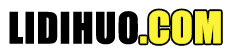# 希尔排序

### 克努特公式

```h = h * 3 + 1
where −
h is interval with initial value 1
```

## Shell 排序如何工作？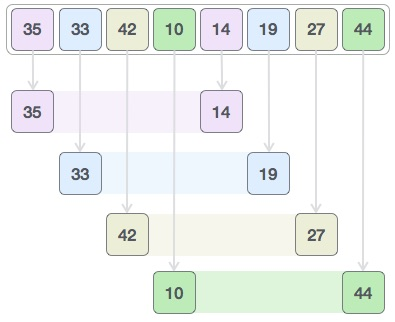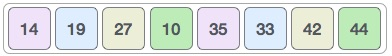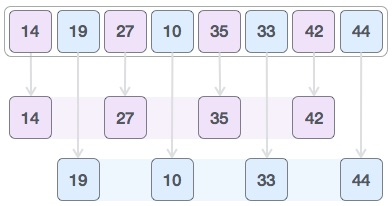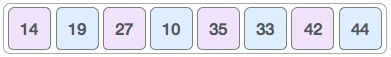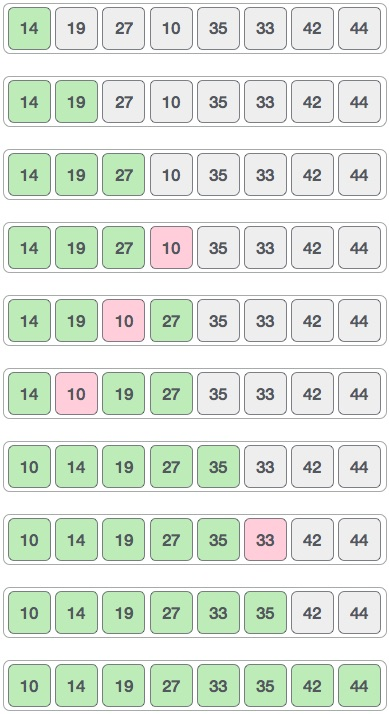### 算法

```Step 1 − Initialize the value of h
Step 2 − Divide the list into smaller sub-list of equal interval h
Step 3 − Sort these sub-lists using insertion sort
Step 3 − Repeat until complete list is sorted
```

## 伪代码

```procedure shellSort()
A : array of items

/* calculate interval*/
while interval < A.length /3 do:
interval = interval * 3 + 1
end while

while interval > 0 do:
for outer = interval; outer < A.length; outer ++ do:
/* select value to be inserted */
valueToInsert = A[outer]
inner = outer;
/*shift element towards right*/
while inner > interval-1 && A[inner-interval] >= valueToInsert do:
A[inner] = A[inner-interval]
inner = inner-interval
end while
/* insert the number at hole position */
A[inner] = valueToInsert
end for
/* calculate interval*/
interval = (interval-1) /3;
end while

end procedure
```